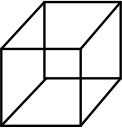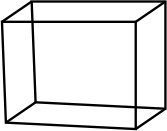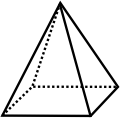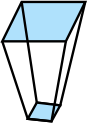# SOF - IMO Level 2 Sample Papers for Class 8

Class 8 sample paper & practice questions for the level 2 of International Mathematics Olympiad (IMO) are given below. Syllabus for level 2 is also mentioned for these exams. You can refer these sample paper & quiz for preparing for the exam.Q.1 Q.2 Q.3 Q.4 Q.5 Q.6 Q.7 Q.8 Q.9 Q.10
 Q.1 (82)2 - (18)2 =? a) 8218 b) 6418 c) 6400 d) 7204
 Q.2 Find the volume of iron required to make an open box whose external dimensions are 36 cm x 25 cm x 16.5 cm, the box being 1.5 cm thick throughout. Assume that 1 cm3 of iron weighs 8.5 grams. a) 3960 cubic cm b) 3690 cubic cm c) 3990 cubic cm d) 3660 cubic cm.
 Q.3 Which of the following expressions gives the products of 7 numbers which have the following properties . The GCD of each pair of numbers in that group is a. The LCM of the 7 numbers is b. a) a⁷ x b b) a⁶ x b c) a⁸ x b d) a⁶ x b²
 Q.4 There are a total 9 chocolates - 3 each in the flavours of mango, banana and grape. There are also 4 children. If each child is allowed to choose their own favourite flavour, what is the probability that all of them will get the flavours of their choice? a) (11/27) b) (25/28) c) (25/24) d) (26/27)
 Q.5 Due to heavy rain, Mother warned Dia not to go in the garden. Instead she conducted an activity to play with the numbers. She asked that if a number is divided by 5, remainder is 1. If the same number is divided by 2, remainder is 0. What should be the last digit of the number? a) 4 b) 6 c) 3 d) 5
 Q.6 Which of the following is not constructed using a ruler and a set square? a) Perpendicular to a line from a point not on it b) Perpendicular to a line at a point on a line. c) A line parallel to a given line through a given point. d) A perpendicular bisector of a line segment.
 Q.7 It has 6 faces which are all squares. It is a prism. It has a cross-section throughout its length. Which among the following figure justifies the condition? a)b)c)d)Q.8 A taxi charges a fare of Rs.1275 for a journey of 150 Km. How much would it charge for a journey of 124 km? a) Rs. 1,054 b) Rs. 1,064 c) Rs. 1,050 d) Rs. 1,014
 Q.9 Usha wants to print some brochures for her business. If she goes for style 1, it will cost her Rs.81 plus 0.15 per brochure. If she goes for style 2, it will cost her Rs.15 plus 0.3 per brochure. For how many brochures will the price for both the style be the same? a) 440 b) 445 c) 455 d) 430
 Q.10 If you subtract a number x from 47 times that number, and then take the square of the difference, what will the result be? a) 2418x² b) 2143x² c) 2148x² d) 2116x²